Related Articles

# Mesh Surface Plot in MATLAB

• Last Updated : 30 May, 2021

Mesh Surface Plot is used to depict f(X, Y, Z) in a three-dimensional space. Matlab allows users to create mesh surface plots using the mesh() method.

Different syntax of mesh() method are:

• Mesh(X, Y, Z)
• Mesh(Z)
• Mesh(___,C)
• Mesh(___, Name, Value)
• S = mesh()
• Mesh(ax,____)

### Mesh(X, Y, Z)

• It plots X, Y, Z on a three-dimensional surface.
• Matrices X, Y are plotted along the x-y plane and plots matrix Z as height above the x-y plane.
• If size(Z) = [m, n], then X should be of the same size as Z or a vector of size n.
• Y should be the same size as Z or a vector of size m.

Example:

## Matlab

 `% Define matrix of size 2*10``Z = [1:10;``    ``1:10];``     ` `% Define vector Y of size 2``Y = [1 2];`` ` `% Define vector X of size 10 ``X = [1:10];`` ` `% Plot mesh surface``mesh(X,Y,Z)`

Output :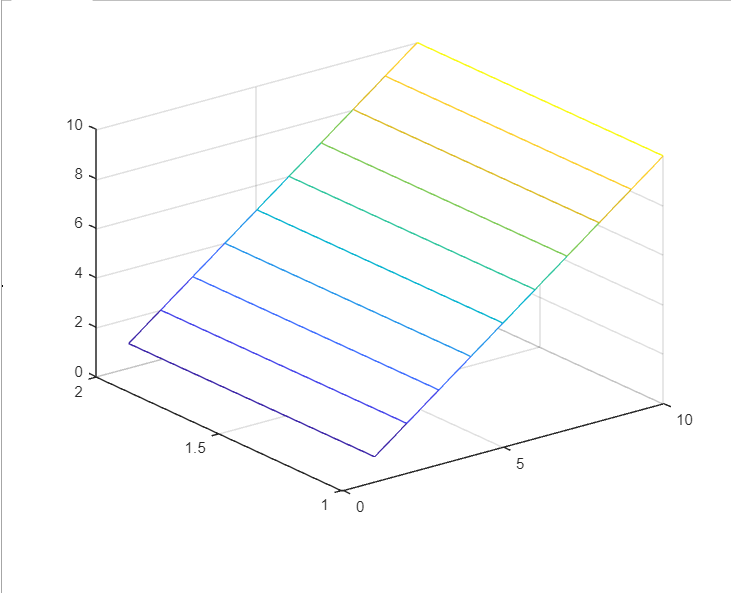### Mesh(Z)

It creates a mesh surface plot with matrix Z by considering column and row indices as x and y-coordinates respectively.

Example:

## Matlab

 `% Creates a random matrix of size 2*10``Z = randi(2,10);`` ` `% Plot a mesh surface``mesh(Z)`

Output :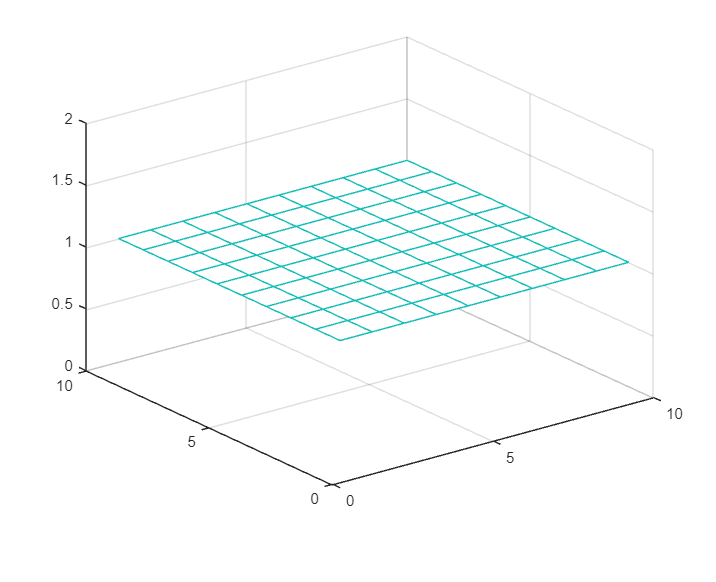### Mesh(___, C)

• It creates a mesh surface plot with the specified color of edges as C.
• C is an m-by-n-by-3 of RGB array where Z is a matrix of size m-by-n.

Example:

## Matlab

 `% Creates a meshgrids X and Y of same size``[X,Y] = meshgrid(2:.7:11);`` ` `% Create matrix Z as same size of X``Z = cos(X)./X;`` ` `% Create a color matrix``C = X.*Y;`` ` `% Plotting mesh surface``mesh(X,Y,Z,C)`

Output :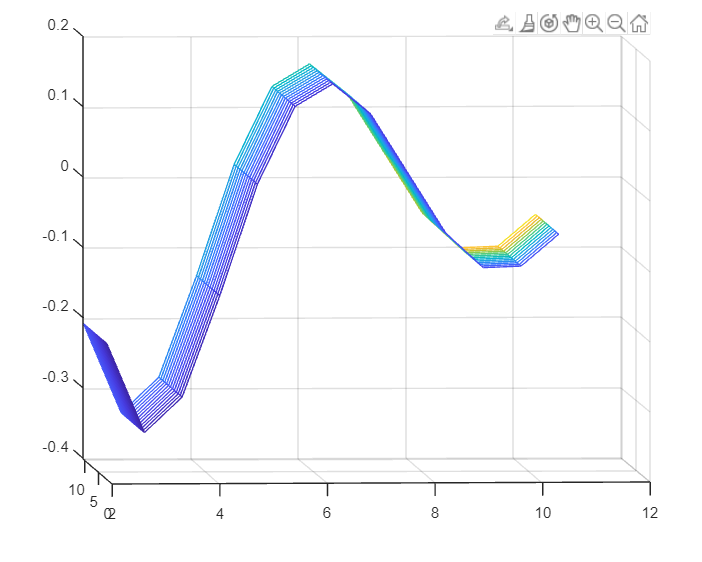### Mesh(___, Name, Value)

• It creates a mesh surface plot with the specified Name-Value pair arguments.
• Some properties are Face Alpha, Edge Color, Line Style etc.

Example:

## Matlab

 `% Create meshgrids X and Y of same size``[X,Y] = meshgrid(4:.2:20);`` ` `% Create matrix Z``Z = X.*Y - sin(X);`` ` `% Create mash plot with FaceAlpha and EdgeColor``mesh(X,Y,Z,``'FaceAlpha'``,``'0.5'``,``'EdgeColor'``,``'flat'``)`

Output :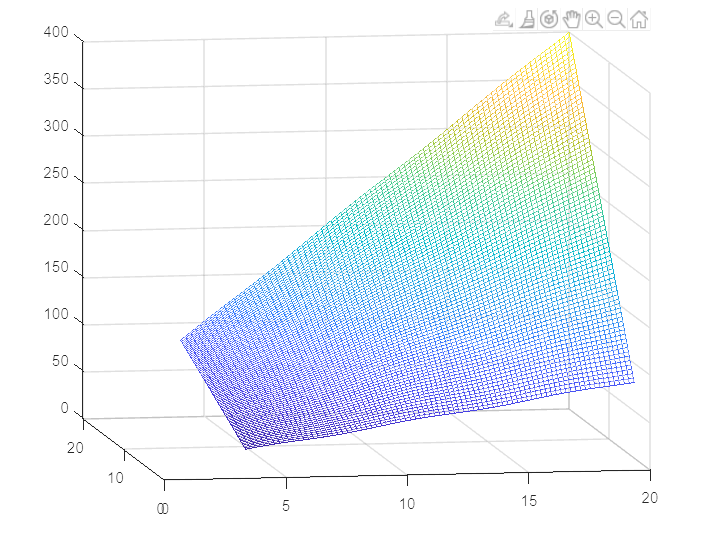### S = mesh(___)

• It creates a mesh plot with specified variables and returns the chart surface objects of the mesh plot.
• You can modify the mesh plot even after creating the plot using the variables.

Example 1:

## Matlab

 `% Creates meshgrids X and Y of same size``[X,Y] = meshgrid(1:.2:3);`` ` `% Initialize Z as of same size of X``Z = X - Y.*X;`` ` `% Plot the mesh plot with X,Y,Z of linestyle = '--'``% We can change surface properties of using variable s``s = mesh(X,Y,Z,``"LineStyle"``,``'--'``)`

Output :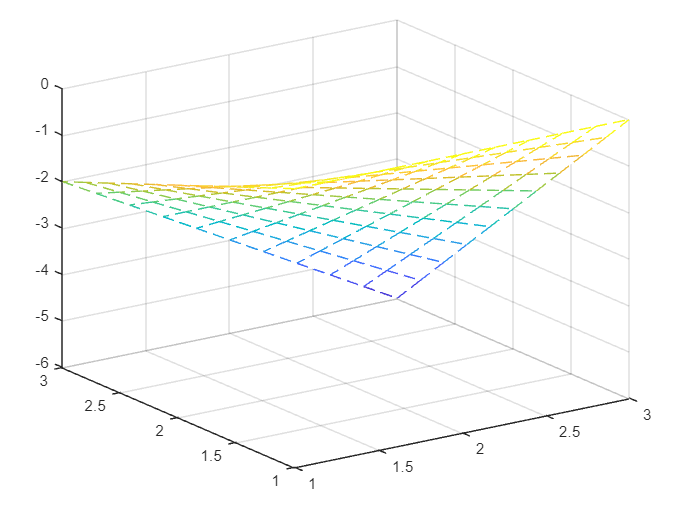Properties of mesh plot :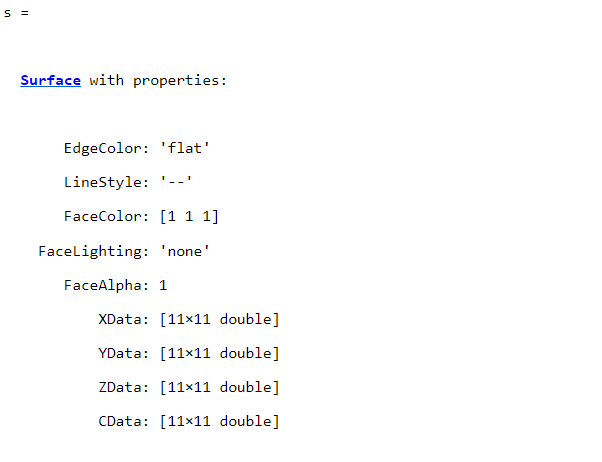Example 2:

## Matlab

 `% MATLAB code for creates meshgrids``% X and Y of same size``[X,Y] = meshgrid(1:.2:3);`` ` `% Initialize Z as of same size of X``Z = X - Y.*X;`` ` `% Plot the mesh plot with X,Y,Z of linestyle = '-' and Facecolor.``s = mesh(X,Y,Z)``s.LineStyle = ``'-'``;``s.FaceColor = ``'[1 0.7 0]'`

Output :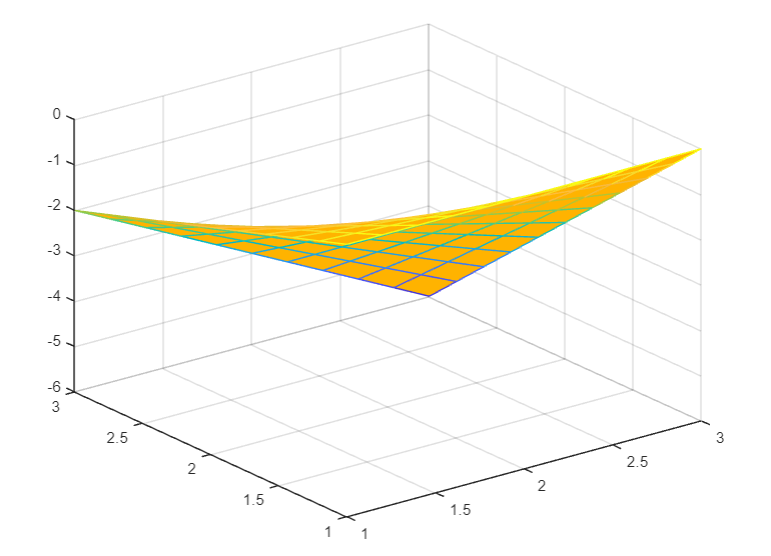### Mesh(ax,______)

This function is used to specify axes in the mesh plot instead of current axes.

## Matlab

 `% MATLAB code for mesh(ax,___) %``[X,Y] = meshgrid(-10:.8:4);``R = sqrt(X.^2 + Y.^2) + eps;``Z = sin(R)./R;``mesh(axes,X,Y,Z)`

Output: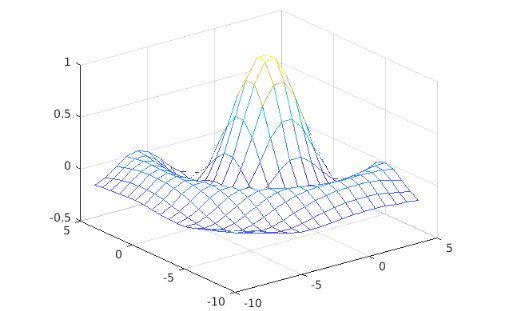My Personal Notes arrow_drop_up